The core math topics covered in grade 4 typically are:

Numbers and Operations

• whole numbers up to the millions
• writing numbers using base 10 and in expanded form
• rounding large numbers to any place
• multiplication of one by 4 digits and 2 by 2 digits
• division of 4 digit numbers by 1-digit numbers
• remainders
• factors and prime factors
• equivalent fractions and comparing fractions
• multiply fractions by a whole number
• converting fractions to/from decimals

Geometry

• area and perimeter of rectangles and irregular rectangular shapes
• points, segments, lines and rays
• identifying, measuring and classifying angles
• perpendicular and parallel lines
• symmetry

Measurement

• converting measurements to different measurement units
• measurement word problems involving distance, capacity, weight, time and money

Data Analysis

• displaying a set of data as a line plot
• analyzing data from graphs

The common core math standards for grade 4 are also worth reviewing.

## Math worksheets for grade 4

Choose from hundreds of free grade 4 math worksheets organized by topic:

### Fractions & decimals

Place Value & Rounding Fractions
Subtraction Decimals
Mental Multiplication

### Other

Multiply in Columns Measurement
Mental Division Geometry
Long Division Factoring
Order of Operations Word Problems
Roman Numerals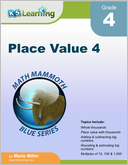Place Value 4 Numbers and place value in the thousands, ten thousands, hundred thousands and millions.  Adding, subtracting, rounding and estimating big numbers.  Multiples of 10, 100 and 1,000.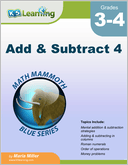Add and Subtract 4 Mental addition and subtraction strategies; adding and subtracting in columns and order of operations.  Also Roman numerals, money problems and bar and line graphs.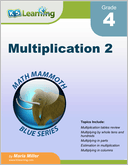Multiplication 2 Multi-digit multiplication including multiplying in parts, multiplying in columns and order of operations.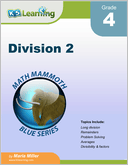Division 2 A continuation of division topics, including long division, averaging and factors.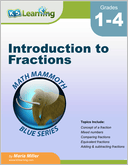Introduction to Fractions Introducing fractions, mostly for grades 3-4. Concept of a fraction, mixed numbers, comparing fractions, equivalent fractions, adding and subtracting fractions and fractions multiplied by a whole number.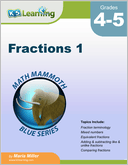Fractions 1 Focus on the addition and subtraction of fractions with like and unlike denominators.  Also mixed numbers, equivalent fractions and comparing fractions.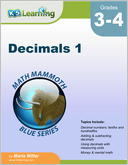Decimals 1 Introduction to decimals (tenths and hundredths). Adding and subtracting decimals mentally and in columns.  Decimals and money.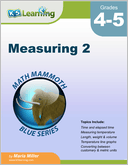Measuring 2 Length, weight, capacity, temperature and time.  Focus on conversion between different measuring units.  Customary and metric units used.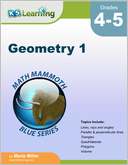Geometry 1 Overview focused on basic plane geometry. Topics include lines, rays and angles; measuring angles; parallel and perpendicular lines, shapes, area, volume. Also rectangular prisms and surface area.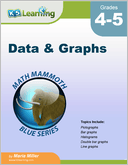Data and Graphs Statistics and common graphs and related topics including pictographs, bar graphs, histograms, double bar graphs, multiple line graphs, circle graphs and mean / median / mode.